Maharashtra State Board course SSC (English Medium) Class 9
Share

# The Ungrouped Frequency Distribution Table of Digits Appearing After the Decimal Point is as Follows: - Algebra

ConceptFrequency Distribution Table

#### Question

The value of π upto 50 decimal places is given below :
3.14159265358979323846264338327950288419716939937510  From this information prepare an ungrouped frequency distribution table of digits appearing after the decimal point.

#### Solution

The value of π upto 50 decimal places is given below :
3.14159265358979323846264338327950288419716939937510
The ungrouped frequency distribution table of digits appearing after the decimal point is as follows:

 Digits Tally Marks Frequency 0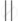2 1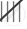5 2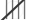5 3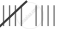9 44 5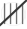5 6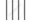4 7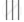3 8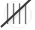5 9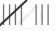8 Total 50
Is there an error in this question or solution?

#### APPEARS IN

Balbharati Solution for Balbharati Class 9 Mathematics 1 Algebra (2019 to Current)
Chapter 7: Statistics
Practice set 7.3 | Q: 6 | Page no. 118
Solution The Ungrouped Frequency Distribution Table of Digits Appearing After the Decimal Point is as Follows: Concept: Frequency Distribution Table.
S